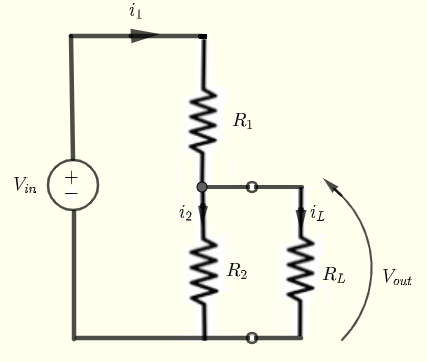# DC Voltage Divider Calculator

An online calculator to calculate the output voltage, current and power of a load in dc voltage divider is presented. Activities using the calculator to investigate the maximum power delivered to the load $R_L$ are suggestsed.## Current, Voltage and Power Formulas

Given $V_{in}$ , $R_1$ , $R_2$ and the load $R_L$, let us find formulas for the current $i_L$ through, the voltage $V_L$ across, and the power delivered to the load (resistor) $R_L$.
Using
Kirchhoff's laws of voltage and current, we can write the following equations:
$V_{in} = R_1 i_1 + R_2 i_2$    (I)
$- R_2 i_2 + R_L i_L = 0$    (II)
$i_1 = i_2 + i_L$    (III)

Substitute $i_1$ in equation (I) by $i_2 + i_L$ to obtain two equations with two unknowns $i_2$ and $i_L$
$V_{in} = R_1 (i_2 + i_L) + R_2 i_2$    (I')
$- R_2 i_2 + R_L i_L = 0$    (II')
Solve the above system of two equations and two unknowns by any method to obtain
$i_L = \dfrac{R_2}{R_L(R_1+R_2)+R_1 R_2} V_{in}$
Use
Ohm's to find $V_L$:
$V_L = R_L i_L = \dfrac{R_2 R_L}{R_L(R_1+R_2)+R_1 R_2} V_{in}$
The power $P_L$ delivered to the load is given by
$P_L = V_L i_L$

## dc Voltage Divider Calculator

Enter the resistances of $R_1$ , $R_2$, $R_L$ in Ohms and the source voltage $V_{in}$ in volts as positive real numbers and press "Calculate". The outputs are the current $i_L$ through $R_L$, the voltage $V_L$ across $R_L$ and the power $P_L$ delivered to the load $R_L$.

 $R_1$ = 10 $\Omega$       $R_2$ = 20 $\Omega$       $R_L$ = 30 $\Omega$ $V_{in}$ = 12 V Decimal Places = 4

## Activities

It can be shown that when $R_L = \dfrac{R_1 R_2}{R_1 + R_2}$, the power delivered to the load $R_L$ is maximum.
Select values for $R_1$ and $R_2$ and calculate $R_L$ using the formula $R_L = \dfrac{R_1 R_2}{R_1 + R_2}$ and use all three values in the calculator above to calculate $P_L$.
Then keep $R_1$ and $R_2$ with the same values selected above but change $R_L$ slightly above or below the calculated value. Which value of $R_L$ gives the maximum value of $P_L$.
Example
Let $R_1 = 20$ and $R_2 = 30$, hence $R_L = \dfrac{20 \times 30}{20 + 30} = 12$
Enter the values $R_1 = 20$, $R_2 = 30$ and $R_L = 12$ in the Calculator.
What is the values of $P_L$?
Keep $R_1 = 20$ and$R_2 = 30$ but change $R_L$ to values different from 12, higher values such as 13, 14, 15, ... and lower values such as 11, 10, 9 ...and take note of the values of $P_L$.
Which value of $R_L$ gives the highest value of $P_L$?